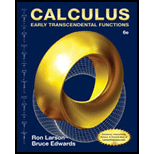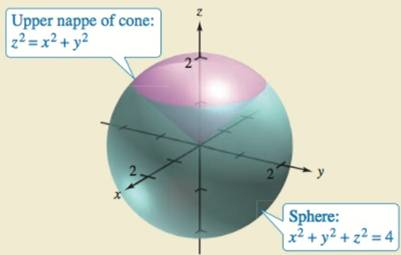Chapter 14.7, Problem 48ECalculus: Early Transcendental Fun...

6th Edition
Ron Larson + 1 other
ISBN: 9781285774770

Solutions

Chapter
SectionCalculus: Early Transcendental Fun...

6th Edition
Ron Larson + 1 other
ISBN: 9781285774770
Textbook Problem

HOW DO YOU SEE IT? The solid is bounded below by the upper nappe of a cone and above by a sphere (see figure). Would it be easier to use cylindrical coordinates or spherical coordinates to find the volume of the solid? Explain.To determine

To Calculate: Whether it would be easier to use cylindrical coordinates or spherical coordinates to calculate volume of the given solid bounded below by the upper nappe of a cone z2=x2+y2 and above by a sphere x2+y2+z2=4 as shown in figure in the question.

Explanation

Given:

The figure:

Refer to figure in question.

The solid is bounded below by the upper nappe of a cone z2=x2+y2 and above by a sphere x2+y2+z2=4.

Formula used:

Volume of a solid in cylindrical coordinates is,

V=QdV=θ1θ2g1(θ)g2(θ)h1(rcosθ, rsinθ)h2(rcosθ,rsinθ)rdzdrdθ

And volume of a solid in spherical coordinates is,

V=QdV=φ1φ2θ1θ2ρ1ρ2ρ2sinφdρdθdφ

Calculation:

It is clear from the figure that z varies from z=x2+y2 (from upper nape of the cone) to z=4x2y2 (surface of the sphere).

Consider the general equation of sphere that is x2+y2+z2=ρ2

Where ρ is the radius of sphere. Therefore, radius of the provided sphere x2+y2+z2=22 is,

ρ=2unit

Now the cone z2=x2+y2 and sphere x2+y2+z2=4 intersects at,

x2+y2+z2=4z2+z2=42z2=4z2=2

Take square root on both the sides,

z=2

It is known that in spherical coordinate z=ρcosϕ.

Where ϕ is the angle made by plane z2=x2+y2 or 2=x2+y2 to make sphere x2+y2+z2=4.

Substitute the value of z and ρ in equation z=ρcosϕ to find the value of ϕ,

z=ρcosϕ2=2cosϕ22=cosϕϕ=arccos(22)

ϕ=π4

Thus, bounds of ϕ is, 0ϕπ4

For sphere angle θ varies from 0θ2π

Hence, volume of provided solid is,

QV=ϕ

Still sussing out bartleby?

Check out a sample textbook solution.

See a sample solution

The Solution to Your Study Problems

Bartleby provides explanations to thousands of textbook problems written by our experts, many with advanced degrees!

Get Started

Find more solutions based on key concepts